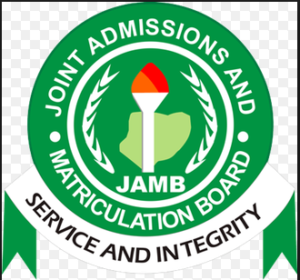# JAMB Mathematics Questions and Answers 2021/2022 To CBT Exams

JAMB Mathematics Questions and Answers 2021/2022 To CBT Exams: This article is made to updates all candidates sitting for the Joint Admission and Matriculation Board Exams 2021 and are equally looking for the current JAMB Mathematics CBT Questions and Answers for 20221. We will, as well, make available other necessary information to guide candidates while the exams, read and follow them accordingly.## JAMB Mathematics Objectives Questions and Answers

The following are JAMB Mathematics Sample Questions and Answers: Make sure you read the passage carefully before attempting the questions. You are expected to take only one option.

1. The sum of two numbers is twice their difference. If the difference of the numbers is P, find the larger of the two numbers
• A. p/2
• B. 3p/2
• C. 5p/2
• D. 3p

2. A binary operation * is defined by a*b = ab+a+b for any real number a and b. if the identity element is zero, find the inverse of 2 under this operation.
• A. 2/3
• B. 1/2
• C. -1/2
• D. -2/3

A cone has a base radius of 4 cm and a height of 3 cm. The area of its curved surface is

• A. 12 pi cm^2
• B. 20 pi cm^2
• C. 24 pi cm^2
• D. 251 pi cm^2

4. The sum of the first five terms 0f the series 4+2+1… is

• A. 12
• B. -12
• C. -6
• D. 31/4

5. If a√5 + b √2 is the square root of 95-30√10, the values of a and b are, respectively

• A. 5, 2
• B. 2, -5
• C. -5, 3
• D. 3, -5

## How to get JAMB Mathematics Answers

If you want to get JAMB Literature Answers, all you have to do is to follow every update on this page. The JAMB Questions are not very far from the previous question. candidates who made use of their past questions and equally practice with the CBT Application will not have anything to worry about. SEE: JAMB Recommended Novel Summary

## How To Answer JAMB Mathematics Questions

To Make the best out of the exams, we encourage candidates to read the questions carefully before taking any option. The idea is that you will understand the questions very well. There are some questions that demand less time while others do not. Calculate your time very well.

## Major Subjects to consider in Mathematics

The following Core Subjects will not fail to appear:

• Number and Numeration
• Algebra
• Geometry / Trigonometry.
• Calculus
• Statistics

SEE:

RECOMMENDED:

## Time Duration for JAMB Mathematics Exams

The time expected to answer Mathematics questions may not be more than 50 minutes. Remember that you will have three subjects to tackle, do not waste your time in one subject. The total time for the three subjects will be within 2 hours 30 minutes.

### JAMB Mathematics Frequently Repeated questions

There are many Questions candidates may not fail to see in Every Year JAMB Exams. These Questions are there for candidates who make good use of their Past Questions and The CBT Practice Softwares.

### Other Factors to consider in Answering Maths Questions

4. Explore Both Sides of an Argument. …

#### Related Searches

jamb 2021 Mathematics questions and answers

jamb past questions and answers on Mathematics

jamb 2020 Mathematics questions and answers pdf

how to pass jamb Mathematics

repeated questions on Mathematics

jamb Mathematics syllabus 2020

jamb Mathematics hot topics